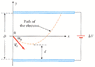# Challenging Question

Touchme

## Homework Statement

An electron is fired at a speed vo = 5.0 106 m/s and at an angle o = -44° between two parallel conducting plates that are D = 3.7 mm apart, as in Figure P16.66. The voltage difference between the plates is V = 100 V.

(a) Determine how close, d, the electron will get to the bottom plate.

## Homework Equations

Conservation of energy:
(1/2)mv^2 = -qEd
E=V/D

## The Attempt at a Solution

I was thinking conservation of energy because the electron is initially moving and when it reaches max, it stops and KE is converted to PE.
(1/2)(9.109e-31)[(5e6)sin(44)]^2 = (-1.602e-19)(27027.02)(d)
d = 0.001269 m

E = (100/0.0037) = 27027.2 N/C

Anyone knows what is wrong?

#### Attachments

•p16-66.gif
8.5 KB · Views: 466
Last edited:

Mindscrape
I would probably tackle it with forces so that the vectors remain intact. You never said what the question was asking for.

Touchme
sry forgot the question. I edited the post. I was thinking of using projectile motion to solve this question, but I can't solve for the force that moves the electron towards the positive plate.
I know F = qE

edit: omg I just told myself how to solve this. Nevermind, I think I know how to do this. :]

Last edited:
obeserabbit
bump

is that the correct method? i tried using it and got an incorrect answer